PSABM5V6 - Practice---Acc7.5 Lesson 8 Slopes Don't Have to be Positive (8.EE.B)
Part A)

Suppose that during its flight, the elevation e (in feet) of a certain airplane and its time t, in minutes since takeoff, are related by a linear equation. Consider the graph of this equation, with time represented on the horizontal axis and elevation on the vertical axis. For the following situation, decide if the slope is positive, zero, or negative:

The plane is cruising at an altitude of 37,000 feet above sea level.

Select one:
Part B)

The plane is descending at rate of 1000 feet per minute.

Select one:
Part C)

The plane is ascending at a rate of 2000 feet per minute.

Select one:
Part A)

A group of hikers park their car at a trail head and hike into the forest to a campsite. The next morning, they head out on a hike from their campsite walking at a steady rate. The graph shows their distance in miles, d, from the car on the day of their hike after h hours.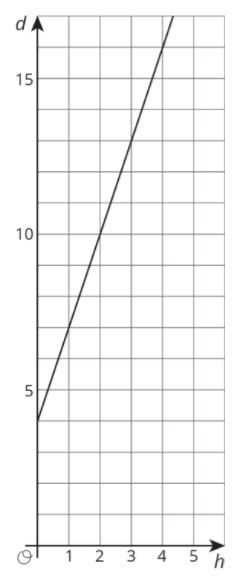How far (in miles) is the campsite from their car?

Type your answer below as a number (example: 5, 3.1, 4 1/2, or 3/2):
Part B)

Explain how you know.

Part C)

Write an equation that describes the relationship between d and h.

Complete the equation below.

d = _______________

Part D)

After how many hours will the hikers be 16 miles from their car?

Type your answer below as a number (example: 5, 3.1, 4 1/2, or 3/2):
Part E)

Elena’s aunt pays her \$1 for each call she makes to let people know about her aunt’s new business.

The table shows how much money Diego receives for washing windows for his neighbors.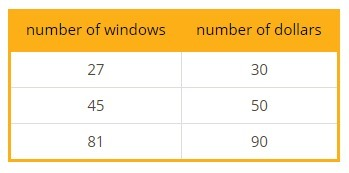Check all the statements about the situation that are true.

Select all that apply:
Part A)

Each square on a grid represents 1 unit on each side.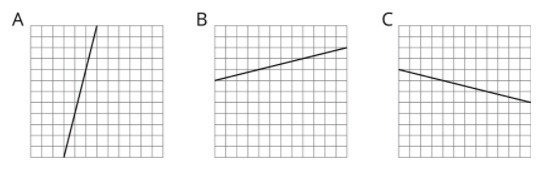Which line has a slope of?

Select one:
Part B)

Which line has a slope of?

Select one:
Part C)

Which line has a slope of 4?

Select one:
Part A)

Priya and Tyler are discussing the figures shown below. Priya thinks that B, C, and D are scaled copies of A. Tyler says B and D are scaled copies of A. Do you agree with Priya, or do you agree with Tyler? Explain your reasoning.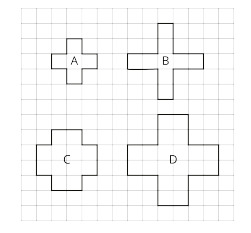Select one:
Part B)

Part A)

Solve each equation, and check your solution.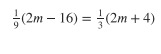m= _______

Type your answer below as a number (example: 5, 3.1, 4 1/2, or 3/2):
Part B)

Solve each equation, and check your solution.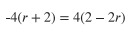r= ________

Type your answer below as a number (example: 5, 3.1, 4 1/2, or 3/2):
Part C)

Solve each equation, and check your solution.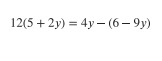y= ________